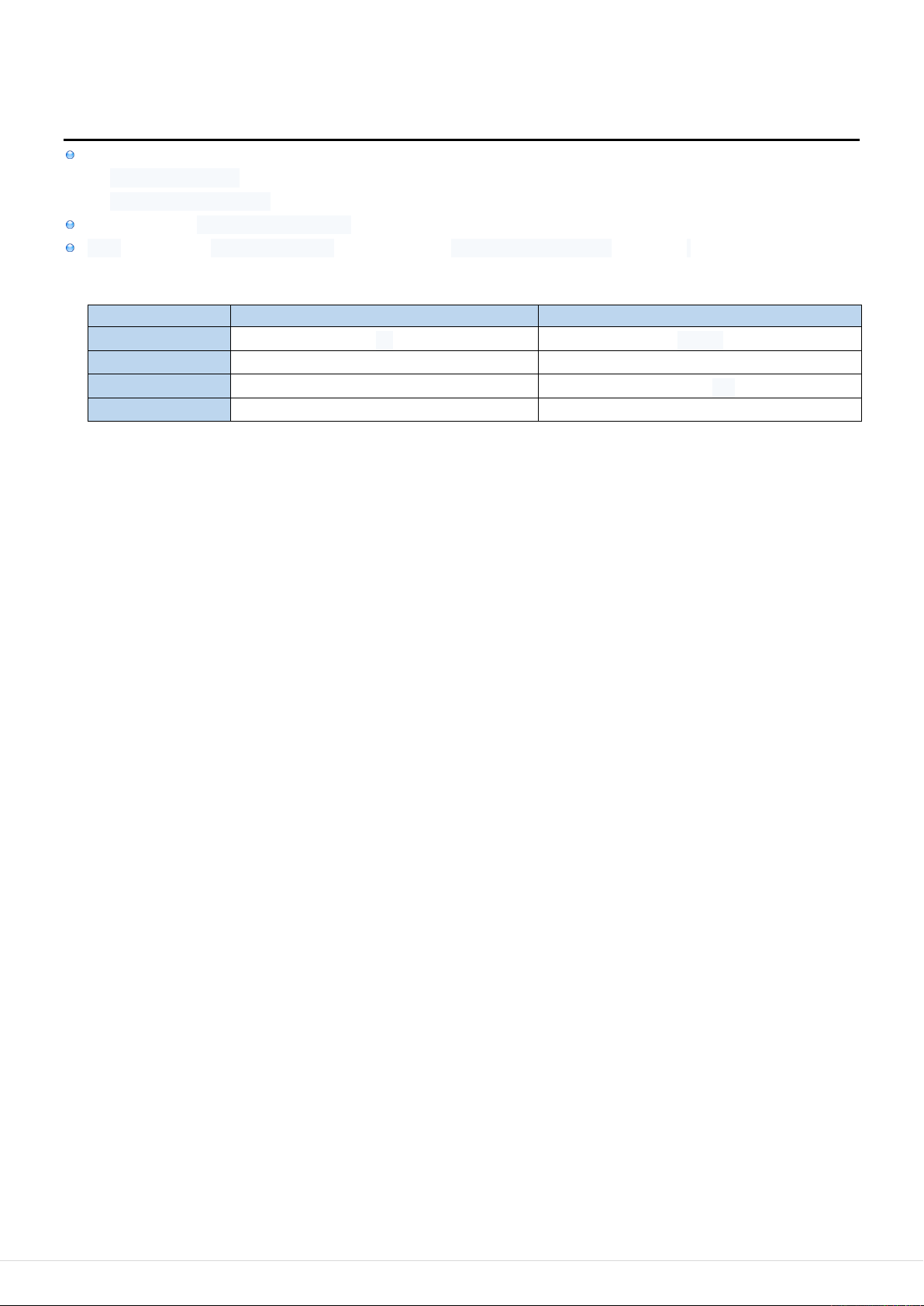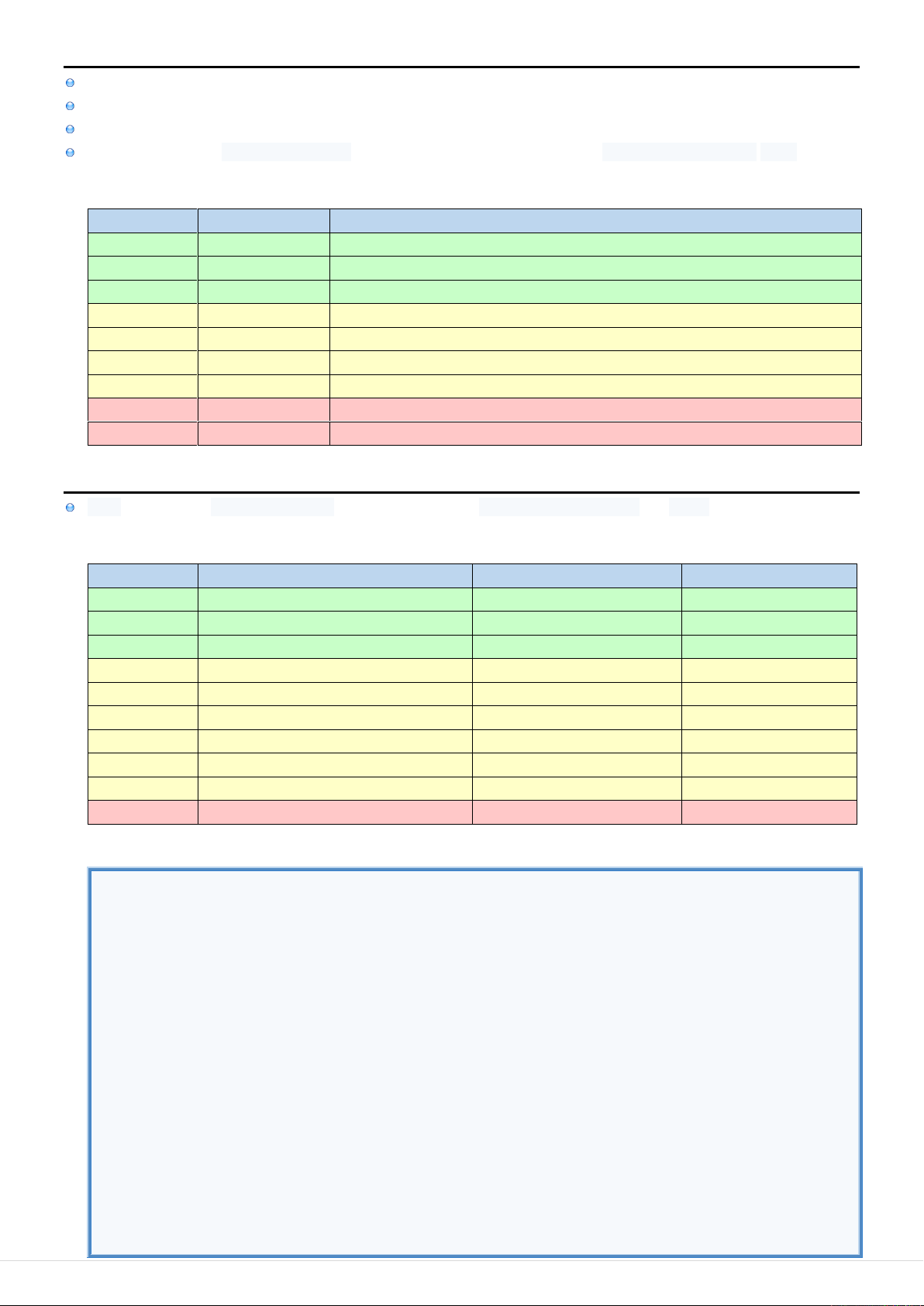JAVA: Summary / Data Types 100
3
3
.
.
3
3
D
D
a
a
t
t
a
a
T
T
y
y
p
p
e
e
s
s
I
I
n
n
f
f
o
o
Java Data Types are grouped into
Primitive Data Types (boolean, int)
Non-Primitive Data Types (each declaration of Class is considered a new Data Type)
String is the only Non-Primitive Data Type which can be defined using Literal (String Literal "Hello").
array is considered Primitive Data Type but it can contain Non-Primitive Data Types (like String).
Primitive vs Non-Primitive Data Types [R]
PRIMITIVE
NON-PRIMITIVE
EXAMPLE
int
Integer
METHODS
Don't have Methods
Have Methods
VALUE
Always has a value
Can be null
NAMING
Name starts with lowercase
Name starts with uppercaseJAVA: Summary / Data Types 101
L
L
i
i
t
t
e
e
r
r
a
a
l
l
s
s
Literal is string representation of both data type and data value: 10, "John", ["AUDI", "FIAT"]
Literal notations are different ways of constructing literals that represent the same data type: 10, +10, 012 or 0xA.
Escape Sequences are used for Character & String Literals to symbolize certain characters that can't be written.
All Literals represent Primitive Data Types (except String Literal which represents Non-Primitive Data Type String).
Literals
LITERAL
EXAMPLE
NOTATIONS
Null
null
Boolean
true, false
Character
'A'
Character: 'A' Code: '\101' Unicode:'\u0041'
Integer
65
Decimal: 65 Hexadecimal: 0x41 Octal: 0101 Binary: 0b1000001
Long
65L
Decimal: 65l Hexadecimal: 0x41L Octal: 0101L Binary: 0b1000001L
Float
65.4F
Basic: 65.4f Scientific: 0.654E+2F
Double
65.4
Basic: 65.4 Scientific: 0.654E+2
Array
{0, 1 , 2 , 3}
String
"Hello \n"
P
P
r
r
i
i
m
m
i
i
t
t
i
i
v
v
e
e
D
D
a
a
t
t
a
a
T
T
y
y
p
p
e
e
s
s
array is considered Primitive Data Type even when it contains Non-Primitive Data Types like String.
Primitive Data Types
EXAMPLE
DESCRIPTION
SIZE
String value = null;
Undefined/unknown value
boolean value = true;
Logical TRUE or FALSE
char value = 'A';
Unicode character
16-bit
byte value = -65;
Signed integer number
8-bit two's complement
short value = -65;
Signed integer number
16-bit two's complement
int value = -65;
Signed integer number
32-bit two's complement
long value = -65;
Signed integer number
64-bit two's complement
float value = 65.4;
Real number
32-bit IEEE 754
double value = 65.4;
Real number
64-bit IEEE 754
int[] value = {1, 2, 3};
Array of integers
Example of Primitive Data Types & Literals
//GENERAL
String value = null; //Nul Literal
boolean value = true; //Boolean Literal: true, false
char value = 'A'; //Character Literal
//NUMBERS
byte value = -65; //Integer Literal: 0x41 0101 0b1000001
short value = -65; //Integer Literal: 0x41 0101 0b1000001
int value = -65; //Integer Literal: 0x41 0101 0b1000001
long value = -65; //Integer Literal: 0x41 0101 0b1000001
float value = 65.4; //Float Literal: 0.654E+2
double value = 65.4; //Float Literal: 0.654E+2
//ARRAY
int[] value = {1, 2, 3}; //Array of int
int[][] value = { {1,2}, {3,4,5} }; //2D Array of int
String[] value = {"John", "Bill"}; //Array of String Objects
//STRING
String value = "Hello \n"; //String Literal. String is NOT Primitive Type.